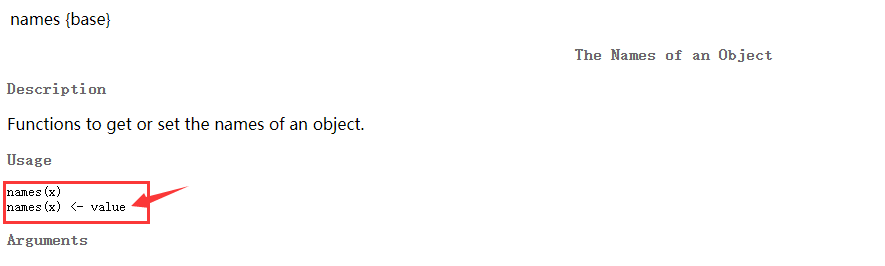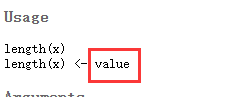• 「杂谈」如何写好R语言apply家族函数
2022-03-28 10:36:39

感觉好久没发有关R语言的博客了。这一年来，我着手开发了两个R包，但基本没怎么发博客。

原因也很简单，可能是我个人的私心吧，或者说是典型的“理论研究者”的“通病”：对于自己感兴趣的东西，不到200%的了解和把握，坚决不分享给别人；一旦分享，力求高质量。

apply家族函数我用了起码也一两年了，现在看起来越来越受欢迎。但它们对初学者来说总不是很友好。

希望这篇po文能对初学者有用。如何衡量有用？就是初学者看完之后就会彻底觉得：但凡是R语言内的循环都能用apply家族的函数解决

# 一、lapply

最好不要试图一开始就用apply函数，因为它比lapply函数复杂。lapply函数是apply家族的函数中逻辑最简单、版本兼容性、最user-friendly的函数。

你甚至可以用lapply这一个函数打天下。

其基本语法也很简单：lapply(Vector, function(ii) { ... })

例如，有这样一个data.frame，它是dat <- data.frame(A=1:5,B=10:14)。我现在要做一件事：对于A列中的每个元素，找出B列中离它最远的那个数（在一维数轴上）。

像这样的问题，让初学者感受到两层循环的压力时，就是apply家族函数大展拳脚的时机。

初学者会这样写：

result <- c()
for(i in 1:nrow(dat)){
alldistance <- abs(dat$A[i]-dat$B) #calculate distances
whichmax <- which(max(alldistance)==alldistance) #which point has the maximal distance
valuemax <- dat$B[whichmax] #value of point with the maximal distance result <- c(result,valuemax) }  准确来说这不是两层循环，而是外层循环，内层向量运算。 但用lapply函数后，就是这样的： result <- lapply(1:nrow(dat),function(i){ alldistance <- abs(dat$A[i]-dat$B) whichmax <- which(max(alldistance)==alldistance) valuemax <- dat$B[whichmax]
return(valuemax)
})


但初学者多少会有些不习惯。

但无论如何，我相信用R的绝大多数人都有一个习惯：把一条命令写出来，马上运行，运行结果正确时，就把这条命令放到脚本里；然后再写下一句命令，再运行，结果正确时再把这个命令塞到脚本里，如此往复循环…

这个习惯同样有利于我们快速地写好lapply函数。

Step1: 写好框架lapply(Vector, function(element) { ... })。其中Vector有两种方式。一种是像上面这个例子那样，用索引；另一种干脆直接遍历元素，比如上面这个例子中lapply的第一个元素变成dat$A Step2: 将循环索引push到工作变量环境中。例如上面这个案例，框架搭好以后，直接在命令行运行i <- 1。接下来编代码就容易了，就像编一个for循环那样，写一句运行一句。 Step3: 返回值。返回任何东西都可以，例如一个标量、向量、数据框等等。一开始接触时建议加return，像我这种老油条必然是懒得加了，因为R语言默认一个函数定义中最后一句话的返回值就是整个函数的返回值。 lapply函数的返回值，永远是个list。因此上述代码的第二个版本中result变量实际上是个list。这个list中的每个组分就是lapply中自定义函数的返回值valuemax。要是valuemax不是个标量，而是个更复杂的东西，比如矩阵，那result这个列表可以更复杂。 # 二、do.call 接下来，自然而然的疑问就是说，既然你lapply函数返回一个list，那我怎样把这个list转换成一个向量，或者数据框呢？ 有这种疑问很正常，因为很多时候我们用一个循环，或者用apply家族函数所得到的最终结果应该是个向量，或者数据框。例如上述案例最终就应该得到一个向量。 怎么办呢？ 如果要得到向量，请用do.call(c, <A list object>) 如果要得到数据框，请用do.call(rbind, <A list object>) 因而do.callapply家族函数往往是联合在一起用的。 result <- do.call(c,lapply(1:nrow(dat),function(i){ alldistance <- abs(dat$A[i]-dat$B) whichmax <- which(max(alldistance)==alldistance) dat$B[whichmax]
}))


# 三、让代码更严谨

上述案例中的代码有个缺陷（尽管影响不大）：lapply函数中的自定义函数内部使用了dat变量，但这个dat变量实际上采用的是函数外部的dat

在一个良好的、可维护的代码中，这样的风范不值得提倡。因此稍作修改：

result <- do.call(c,lapply(1:nrow(dat),function(i, dat){
alldistance <- abs(dat$A[i]-dat$B)
whichmax <- which(max(alldistance)==alldistance)
dat$B[whichmax] }, dat = dat))  请注意，如果不改成这样的形式，在R包编译的时候既不会报WARNING，也不会报NOTE，但万一程序一复杂，计算结果不对的话，这种不规范只会导致代码检查的工作量增大。 # 四、apply家族的其他函数 我单方面认为，综合普适性、用户友好性和实用性，给apply家族排个名，其次序是这样的：lapply>sapply>apply>tapply lapply不多说了，万能的，并且关键是版本兼容性好。啥叫版本兼容性？那我就先来吐槽下sapplyapply的坑爹之处。 sapplylapply极其相似，但有两处不同：① 函数的第一个参数不是个向量，而是个列表，仅此而已；② sapply函数会自动评估返回值是否能合并成一个向量，或者一个数据框；能合并的，就尽量合并；不能合并的，跟lapply函数一样以列表的形式返回。 ②这种画蛇添足的功能，在我看来就是搞事情。 case1 <- sapply(as.list(1:4),function(xx){ if(xx==3) rep(999,2) else xx }) case2 <- sapply(as.list(5:8),function(xx){ if(xx==3) rep(999,2) else xx })  运行代码可知，case1是个列表，case2却是个向量。那么，在真正开发软件时，你究竟要不要在外面加一个do.call呢？加，那case2就直接报错；不加，那case1case2根本不是一种数据类型，下游计算仍要出问题。 幸运的是，sapply函数提供了simplify这个参数。该参数缺省值是TRUE，表示启动②这个功能。你可以将它设置为FALSE，即可关闭它，免得它惹是生非。 apply函数的第一个参数无非是变成了一个矩阵或数据框。使用时，多一个参数MARGINMARGIN = 1代表对每一行施加某一函数；MARGIN = 2代表对每一列施加某一函数。说白了，前者就是以每行为单位搞个循环，后者是以每列为单位搞个循环，没什么新奇的。apply函数跟sapply有一样的毛病，即会自动评估返回值是否要合并成一个向量或者数据框。好在sapply函数还给你simplify这个选项，让你关掉。但apply函数却不给你这个选项。前几个月还有的，到了R 4.0.4这个版本后，apply函数的simplify选项就已经被搞没了，这点让我很困惑，不知道R Core Team是什么想法。 总之，要开发软件包的话，没啥大事情少用apply吧。 tapply也是一样，会自动评估并合并，但好在给了simplify参数。tapply函数的语法是tapply(X, INDEX, function(xx) { ... })，向量X是数据，INDEX相当于是个label，把数据X的每个元素打上分组标签。后面的自定义函数就是对每个分组标签开展一次apply。这种情况的使用场合本来就比lapply少多了，因此其重要性也很次。开发语言 数学 更多相关内容 • Note: 旧的wordpress博客弃用，于是将以前的笔记搬运回来。 Foreword: We use the operator ...函数f:R→Rf: R \rightarrow Rf:RR称为偶函数，如果对所有x∈Rx \in Rx∈R均有f(−x)=f(x)f(-x) = f(x)f(−x)=f(x... Note: 旧的wordpress博客弃用，于是将以前的笔记搬运回来。 Foreword: We use the operator “ ⊕ \oplus ” to express “direct sum”. Question: 函数 f : R → R f: R \rightarrow R 称为偶函数，如果对所有 x ∈ R x \in R 均有 f ( − x ) = f ( x ) f(-x) = f(x) 。函数 f : R → R f: R \rightarrow R 称为奇函数，如果对所有 x ∈ R x \in R 均有 f ( − x ) = − f ( x ) f(-x) = -f(x) 。用 U e U_e 表示 R R 上的实值偶函数的集合，用 U o U_o 表示 R R 上实值奇函数的集合，证明 R R = u e ⊕ U o R^R = u_e \oplus U_o . Solution: Let U e = { f : R → R U_e = \{f: \mathbb{R} \rightarrow \mathbb{R} : such that f is even } \} , U o = { f : R → R U_o = \{f: \mathbb{R} \rightarrow \mathbb{R} : such that f is odd } \} . For each function f ( x ) ∈ R R f(x) \in \mathbb{R}^\mathbb{R} , we can divide it into: f e ( x ) = f ( x ) + f ( − x ) 2 f_e(x) = \frac{f(x) + f(-x)}{2} , f o ( x ) = f ( x ) − f ( − x ) 2 f_o(x) = \frac{f(x) - f(-x)}{2} . Clearly f ( x ) = f o ( x ) + f e ( x ) f(x) = f_o(x) + f_e(x) . Therefor R R = U e + U o \mathbb{R}^\mathbb{R} = U_e + U_o . Since U e ∩ U o = f 0 ( x ) = 0 U_e \cap U_o = f_0(x) = 0 , R R = U e ⊕ U o \mathbb{R}^\mathbb{R} = U_e \oplus U_o . 展开全文• 二、具体看看傅里叶的庐山真面目 首先明确的是f(t)是实函数，才可进行以下的推到—— 三、结论与总结 实部R(w)是偶函数，虚部是奇函数。 F(w)的模： 所以说模是w的偶函数。 相位角： 所以说相位角是w的奇函数。 这... ### 许多人在学习信号与系统的时候，是不是会碰到许多疑惑，其实并不是特别需要你去解决，但是作为一个热爱数学的工科生来说，不解决的话内心真的可能是挣扎。 傅里叶变换中说F(w)的模是偶函数，相位是奇函数，我们一般都是直接去接受的。那么这些具体来说是怎么得到的呢，请继续往下看看吧！ ### 一、认识一下傅里叶的正变换 这是傅里叶变换的标准形式，说实话，从这里直接去观察它模的奇偶我是做不到的！ ### 二、具体看看傅里叶的庐山真面目 首先明确的是f(t)是实函数，才可进行以下的推到—— ### 三、结论与总结 ### 实部R(w)是偶函数，虚部是奇函数。 F(w)的模： 所以说模是w的偶函数。 相位角： 所以说相位角是w的奇函数。 这就是所有的内容，希望对大家有所帮助！展开全文idea • 总所周知，R语言有些神奇的赋值操作， 同一个函数既可以正常使用获取对象属性， 又可以给返回值赋值从而修改原对象的属性 names 下例实现了给对应元素一次性赋值name属性的功能。 > a = c(1,2) > names(a) = c... ## 前言 总所周知，R语言有些神奇的赋值操作， 同一个函数既可以正常使用获取对象属性， 又可以给返回值赋值从而修改原对象的属性 names 下例实现了给对应元素一次性赋值name属性的功能。 > a = c(1,2) > names(a) = c("张三","李四") > names(a)  "张三" "李四" > a 张三 李四 1 2 >  length 下例：length被主动赋值一个更小的数，实现了元素删除的效果。 > a 张三 李四 1 2 > length(a)  2 > length(a) = 1 > a 张三 1 > length(a)  1  虽然这并不符合常规的编程思想（至少我觉得很奇怪），但是这一功能确实简化了R语言使用者的操作，让精力真正放在统计工作当中。 那么问题来了： R语言开发者，如何利用这一特性，帮助自己实现类似方法呢？ 这个时候老师给我们提出一个问题 我们要如何实现一个函数first(a) 这个函数有两种用法 ①first(a) 返回a的第一个元素 ②first (a)<- value 将value赋值给a的第一个元素(覆盖) ### 一、直接尝试 我们先定义一个简单的first函数，获取第一个元素 > first = function(x)x > x = c(1,2,3,4) > first(x)  1  然后我们仿照names的方式，尝试直接给first(c(1,2,3))赋值 > first(x) = 6 Error in first(x) = 6 : 没有"first<-"这个函数  显然，没有成功，但是其报错提示给了我一个线索"first<-“函数 我定义的函数确实不叫"first<-”，因此我猜测，如果需要对R语言函数的返回值赋值，需要先创建其对应的"函数名<-"函数 ### 二、验证猜测 对于"first<-"这个函数，我猜测其函数功能就是将等号右侧的值当作参数传给函数并且实现赋值。 因此我构造如下方法： > first<- = function(x,a){x=a;x} 错误: 意外的'='在"first<- ="里  显然 <- 和 = 混用，R语言解释器无法正常理解这条语句 因此我想到用()、’’、""将first<- 分隔开，重新定义。 > (first<-) = function(x,a){x=a;x} 错误: 意外的')'在"(first<-)"里 > 'first<-' = function(x,a){x=a;x} > "first<-" = function(x,a){x=a;x} >  显然，单引号、双引号都起到了隔离的作用，于是我开始验证其作用 > first(x) = 5 Error in first<-(*tmp*, value = 5) : 参数没有用(value = 5)  对于这个返回结果我感觉程序已经调用了"first<-"这个函数，但是还有什么地方出了问题。 找了许久，后面在参考names、length的说明文档中以及其报错信息中找到了灵感参数没有用(value = 5)  ## 三、解决方案 我传给这种特殊方法的参数值名称为value，但是我调用的时候只调用了自己定义的a参数，因此仅需将a参数改成value即可。 "first<-" = function(x,value){x=value;x}  完整代码演示： > first = function(x){x} > "first<-" = function(x,value){x=value;x} > x = c(1,2,3,4) > first(x)  1 > first(x) = 5 //给函数返回值赋值 > first(x)  5 //赋值成功 > x  5 2 3 4  ## 四、后记 解决这个问题的过程中，发现网上对于R语言的资料远不如Java、Python等语言。像R语言这样类似的细节问题更难找到解决方案，在今后的学习过程中，还需要多多实践，如果能多看看官方文档也许能更系统的学习。 展开全文• 今天的帖子，是对在R语言里面批量操作的总结：事情的起源来自于临床师弟的需求##读入数据因为excel的使用习惯，很多临床医生收集标本的时候，喜欢合并把同一个患者的信息，只写一次。但是，如果想要更好地分析，我们... • sinx的平方是偶函数，f(-x)=sin²(-x)=(-sinx)²=...(1) 两个偶函数相加所得的和为偶函数(2) 两个奇函数相加所得的和为奇函数(3) 一个偶函数与一个奇函数相加所得的和为非奇函数与非偶函数(4) 两个偶函数相乘所得的... • 多种核函数分类及参数优化 分类数据集采用iris，先用ggplot画出其分布情况，这里用了花萼宽度和花瓣长度作为输入，标签是花的种类 library(ggplot2) ggplot(iris,aes(x=Sepal.Width,y=Petal.Length,col=Species))...支持向量机 • ## 神奇的伽玛函数 千次阅读 多人点赞 2020-01-09 09:32:09 伽玛函数（Gamma函数），也叫欧拉第二积分，是阶乘函数在实数与复数扩展的一类函数。伽玛函数在分析学、概率论、偏微分方程和组合数学中有重要的应用。 我们通常看到的伽玛函数是这样的： 这到底是个什么...公式推导 • 《原函数和导函数的关系》由会员分享，可在线阅读，更多相关《原函数和导函数的关系(7页珍藏版)》请人人文库网上搜索。1、课题：探究原函数与导函数的关系首师大附中 数学组 王建华设计思路这节课是在学完导数和... • ## 函数 千次阅读 多人点赞 2021-02-17 18:49:33 文章目录函数函数函数性态1.单调性2.奇偶性3.周期性4.有界性题型 函数性态 函数 复合函数：如果内层函数的值域与外层函数的定义域的交集非空，这两个函数才能复合，否则不能复合。 反函数函数y=f(x)y=f(x)y=f(x...数学 • 一. R语言绘图系统 R 里，主要有两大底层图形系统，一是 base 图形系统，二是 grid 图形系统。lattice 包与 ggplot2包正是基于 grid 图形系统构建的，它们...python 数据可视化 机器学习 • 是用R语言还是用Python语言？这是一个旷日持久的争论。此，我们可以尝试采用折中路线：创建一个Python脚本，用该脚本模仿R风格的函数，来方便地进行统计！简介用R语言还是用Python语言？这是数据科学和机器学习的... • 转自： ... 一、开篇 数学爱好者们汇集网络论坛的一大乐事就是对各类和数学相关的事物评头论足 • 本文含有：向量的创建与运算两个方便创建向量的函数：rep()与seq()如何访问向量中的元素首先先明白几个要点：R区分大小写，向量A和向量a是两个不同的向量；R可以识别负数，如5+-2可以输出结果3R是计数时是1-base，也... • } =ln{1/[x+√(1+x²)] =-ln[x+√(1+x²) =-f(x) 因为x+√(1+x²)>0恒成立 所以定义域R，关于原点对称 所以是奇函数 扩展资料 判定函数奇偶性的方法： 奇函数在其对称区间[a,b]和[-b，-a]具有相同的单调性，即已知... • 这一份笔记follow了两本非常优秀的R语言教材，分别是Robert I.Kabacoff的《R语言实战》和Peter Dalgaard的《R语言统计入门》，两本教材的思路有所不同，我会根据我自己的思路尽量降低学习门槛，综合来写教程。... • 上次我们提到了R语言殿堂级人物Hadley Wickham，除了耳熟能详的‘ggplot2’ 软件包以外，他还有很多优秀的作品，比如本文将要讨论的‘purrr’ 软件包中的功能强大的map函数。铁哥真诚希望大家能够理解R语言中“函数... • p<-ggplot(data,aes(x=...那我就不问网友问R自己吧。 直接data$sequence data$sequence 然后按tab，自动补齐变量名。来吧，告诉我你是怎么处理这个空格的。 data$sequence type 好的反引号。轻松加愉快 一
• python中有一个高级函数叫做map() 引用廖雪峰的讲解 如果你读过Google的那篇大名鼎鼎的论文“MapReduce: Simplified Data Processing on Large Clusters”，你就能大概明白map/reduce的概念。 我们先看map。map()...
• &#13; &#13; &#13; &#13; &#13; &#13; &#13; 点击蓝字关注这个神奇的公众号～作者：赵镇宁 R语言中文社区特约作者 当考察多个变量间的相关关系时，通...
• 课题：探究原函数与导函数的关系首师大附中 数学组 王建华 设计思路这节课是在学完导数和积分之后，学生从大量的实例中对原函数和导函数的关系有了一 定的认识的基础展开教学的。由于这部分内容课本没有，但数学...
• 满意答案mxd_joan2013.12.02采纳率：50%等级：11已帮助：3101人即f(x,y)=i(x,y).r(x,y)i(x,y)为照明分量(入射分量)，是入射到景物的光强度；r(x,y)为反射分量，是受到景物反射的光强度。具体步骤如下：(1)先对式...
• 前面，本文介绍的是grid包函数在ggplot2作图时的用处。由于要手动调整要素位置，要是你的需求是要业务化的作图，这篇文章可能没什么帮助。本次记录的是一个很神奇的需求，有3张数据来源完全不同，theme什么的一...
• 请问下我的问题哪里呀，我试了好几次，为啥会运行不了啊，看了很多遍
• 第一章 函数 极限 连续 第一节 函数 一. 函数的概念及常见函数 1. 函数概念 函数的两个基本要素：对应关系、定义域 判断两函数相等：从函数的两基本要素入手，即两函数的对应关系（表达式）、定义域相同 对于任意...
• 一、相关函数最大值、 1、自相关函数最大值、 2、互相关函数最大值、 二、能量有限信号的相关函数在 m 趋近无穷时为 0数字信号处理
• 作业5(1)8 5(1) ...令g(x)=1g(x)=1g(x)=1 ，f(x)g(x)=x(1−x)\frac{f(x)}{g(x)}=x(1-x)g(x)f(x)​=x(1−x) ，这个函数在x=0.5处取得极大值0.25 。我们首先生成一个服从（0,1）区间的均匀分布的随机数u1
• 模块化编程定义：把程序进行封装函数封装面向对象面向文件函数：封装完不会执行，调用来使用。函数是什么：一个具有特定功能的代码块函数的作用：封装代码，提高代码的重用性；提高开发效率；降低后期维护成本。函数...
• ## 傅里叶变换

千次阅读 2019-12-09 22:44:21
4.9 奇函数和偶函数的傅里叶变换 4.10 帕塞瓦尔定理 1. 从傅里叶级数到傅里叶变换 首先，我们先回顾一下傅里叶级数的两种形式： 三角形式 指数形式 接着，我们需要了解帕塞瓦尔定理，根据这个定理...
•python...